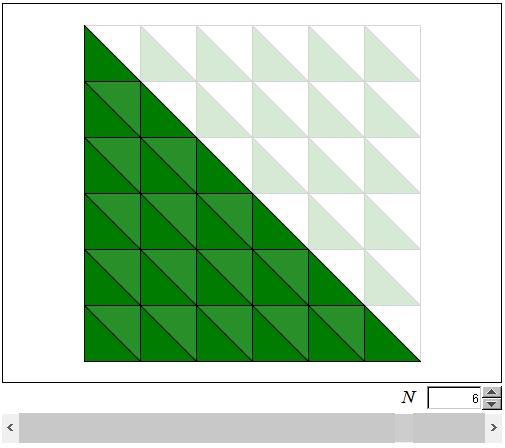# Counting Triangles

The formula

 (1) 1 + 3 + 5 + ... + (2N - 1) = N²

says that the sum of the first N consecutive odd numbers equals N². An elementary proof of (1) could be easily carried out by induction:

For N = 1, the result is immediate. Assume (1) holds for N = k:

 (1') 1 + 3 + 5 + ... + (2k-1) = k²,

and consider (1) for N = k+1:

 (1'') 1 + 3 + 5 + ... + (2k-1) + [2(k+1)-1] = (k+1)².

The left hand side of (1'') differs from the left hand side of (1') by exactly one terms, viz., the last one:

 [2(k+1)-1] = 2k + 1.

The difference of the right hand sides is exactly the same:

(k+1)² - k² = 2k + 1.

Thus (1'') follows from (1'), which completes the induction proof.

There are several visual proofs - Proofs Without Words - of this fact. The applet below purports to present another one. It also hints at a closely related but seldom mentioned result.

### If you are reading this, your browser is not set to run Java applets. Try IE11 or Safari and declare the site https://www.cut-the-knot.org as trusted in the Java setup.What if applet does not run?

For both extreme positions of the slider knob at the bottom of the applet, the number of dark green triangles is exactly the same. The configurations, however, are different.

At the outset, with the nob at the extreme right, we see an isosceles right triangle subdivided into smaller triangles by the auxiliary lines parallel to the sides of the triangles. The lines are drawn through the points on the sides of the triangle that divide the sides into N equal parts. How many small triangles are there? The answer, Exactly N², seems unexpected to many. The effect is often amplified by a prior reference to subdivision of a square into smaller squares using the same procedure: divide the sides of a square into N equal parts and through the division points draw lines parallel to the other sides of the square. As every one knows, this procedure yields N² small squares. How can it be that for squares and triangles the procedure leads to the same number: N²? The applet tries to illustrate exactly this fact.

When the slider knob is in the leftmost position, the applet shows a square divided into smaller squares, each of which is further divided into two small triangles by its diagonal. One of the triangles is color green. Thus, this is obvious that the number of green triangles in the latter configuration is the same as the number of small squares.

When the knob moves from right to left, some triangles in the original configuration fade into background. Simultaneously, the same number of small triangles emerge in the upper right part of the square. Had the second configuration been drawn on a piece of paper, it would have been possible to fold the big square along its main diagonal. The emerging triangles would then appear to overlap the fading triangles. So that indeed the number of the small triangles is the same in both configurations. And since, in the second (leftmost) configuration, the number of small triangles equals the number of small squares, we conclude that the number of the triangles in the first (rightmost) configuration is N².

One can easily observe the relevance of this fact to (1). The diagonals divide the triangles in the first configuration into groups each containing an odd number of triangles. The number of triangles in any two consecutive groups differ by 2. There is 1 triangle in the first (lower left) group, 3 in the next one, 5 in the second, and so on. The last group directly under the main diagonal of the square contains (2N - 1) small triangles. So we get an additional proof of (1).

But the applet suggests yet another, more algebraic in nature, proof. Consider the second configuration. Using the formula for triangular numbers, we see that under the main diagonal there are

 (2) 1 + 2 + 3 + ... + N = N·(N + 1)/2

green triangles. Their number above the diagonal equals

 (2') 1 + 2 + 3 + ... + (N - 1) = (N - 1)·N/2.

Adding the two together we obtain

N·(N + 1)/2 + (N - 1)·N/2 = N²,

which is the basis to a well known proof without words. (The fact that the 2-color checkerboard pattern splits the set of all triangles unevenly has an interesting repercussion.)

Finally, there is an even simpler proof entirely based on geometric considerations. If a square is subdivided into N² small squares, each of which is further split into 2 triangles, then the total number of triangles is 2N². One half of the square surely contains just half of this amount: 2N²/2 = N².

### References

1. R. B. Nelson, Proofs Without Words, MAA, 1993, pp. 71-73•